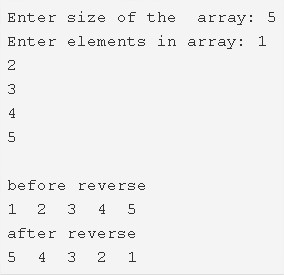# C Program To Find Reverse Of An Array – C Programs

C program to find the reverse of an array – In this article, we will explain the many means to find the reverse of an array in C programming.

Suitable examples and sample programs have also been added so that you can understand the whole thing very clearly. The compiler has also been added with which you can execute it yourself.

The means used in this piece are as follows:

• Using Standard Method
• Using Function

As it is very well known, an array is a sequential arrangement of a bunch of elements in a horizontal fashion. The locations of the elements of an array are defined by pointers.Firstly, as per the given example above, you need to specify the size of the array.

In this case, the size of the array in the example is chosen to be 5.

Then, you need to enter the elements into the array.

The elements entered in this array are as follows:

1 2 3 4 5

The array after reversing the elements becomes like this:

5 4 3 2 1

Thus, the many ways to do the same in C programming are as follows:

## Using Standard Method

1. Read the size of the array and initialize to the variable n.

2) scanf() function reads the entered element and will assign the element to a[i] as scanf(“%d”,&a[i]) using for loop for(i=0;i<n;i++).

3) The main() function calls the print functoion by passing array a,size of the array as arguments. The print() function prints the arrat elements using for loop for(i=0;i<n;i++) as prinf(“%d”,a[i]).

4) To reverse the array element

for loop from i=0 to i<n/2

a) assign the first element with index ‘i’ to temp.

b) assign last element with index [n-i-1] to first element with index ‘i’.

c) assign temp to last element with index [n-i-1]. Repeat these a,b,c steps by increasing i value until i<n/2.

In the above steps, we interchanged the first half of the array elements with 2nd half array elements from the last index.

5) After reversing the given array main() calls the print() function to print the reverse array.

Output:

### Using Function

1. The function reverse() contains the code to reverse the given array.

2) The main() function calls the print() function before reversing the array. The function print() prints the given array element using printf statement and the for loop.

3) The main() function calls the reverse() function by passing the given array, size of the array as arguments. The reverse() function

The reverse() function,

a) Assign the 1st element to temp,

b) Assign the last element to 1st and

c) The temp is assigned to the last element

until all iterations of  for loop for(i=0;i<n/2;i++).

4) After all iterations of for loop, we will get the reverse array. The main() function calls the print() function to print the reverse array.

Output:

x

## C Program To Count The Total Number Of Notes In A Amount | C Programs

C program to count the total number of notes in a given amount – In ...